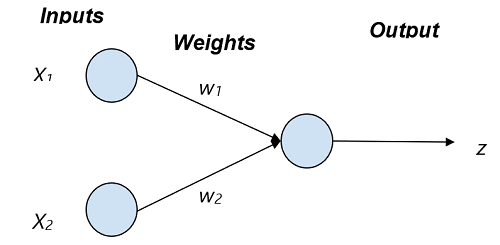# Introduction to Simplest Neural Network | Linear Algebra using Python

Linear Algebra using Python | Introduction to Simplest Neural Network: Here, we are going to learn about the simplest neural network, input and output nodes, related formulas and their implementations in Python.
Submitted by Anuj Singh, on May 23, 2020

A neural network is a powerful tool often utilized in Machine Learning because neural networks are fundamentally very mathematical. We will use our basics of Linear Algebra and NumPy to understand the foundation of Machine Learning using Neural Networks. Our article is a showcase of the application of Linear Algebra and, Python provides a wide set of libraries that help to build our motivation of using Python for machine learning.

The figure is showing the simplest neural network of two input nodes and one output node.Simplest Neural Network: 2 Input - 1 Output Node

Input to the neural network is X1 and X2 and their corresponding weights are w1 and w2 respectively. The output z is a tangent hyperbolic function for decision making which have input as sum of products of Input and Weight. Mathematically,

```    z = tanh(X1w1 + X2w2)
```

Where, tanh() is an tangent hyperbolic function because it is one of the most used decision making functions.

So for drawing this mathematical network in a python code by defining a function neural_network( X, W). Note: The tangent hyperbolic function takes input within range of 0 to 1.

Parameter(s):

```    Vector X  = [[X1][X2]] and W = [[w1][w2]]
```

Return value:

A value ranging between 0 and 1, as a prediction of the neural network based on the inputs.

Application:

1. Machine Learning
2. Computer Vision
3. Data Analysis
4. Fintech
```# Linear Algebra and Neural Network
# Linear Algebra Learning Sequence
# Simplest Neural Network for 2 input 1 output node

import numpy as np

# Use of np.array() to define an Input Vector
V = np.array([.323,.432])
print("The Vector A : ",V)

# defining Weight Vector
VV = np.array([.3,.63,])
print("\nThe Vector B : ",VV)

# defining a neural network for predicting an
# output value
def neural_network(inputs, weights):
wT = np.transpose(weights)
elpro = wT.dot(inputs)

# Tangent Hyperbolic Function for Decision Making
out = np.tanh(elpro)
return out

outputi = neural_network(V,VV)

# printing the expected output
print("Expected Output of the given Input data and their respective Weight : ", outputi)
```

Output:

```The Vector A :  [0.323 0.432]

The Vector B :  [0.3  0.63]
Expected Output of the given Input data and their respective Weight :  0.35316923056117167
```

Preparation

What's New

Top Interview Coding Problems/Challenges!